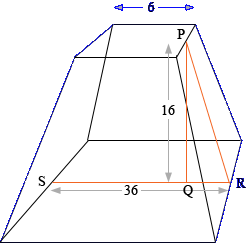SEARCH HOMEMath Central Quandaries & QueriesHello, I am planning to build an open ended, flat-topped pyramid with a rectangular base 28 x 36 in.; a square top 6 x6 in.; and a vertical height of 16 in. I know how to calculate the dimensions of each of the pyramid sides but I don't know how to calculate the interior angles when I bend the sheet metal. I will cut the four sides separately and add a one inch flange to each "vertical" edge of the two larger sides to allow for a bonding surface for the assembly. How do I calculate that angle? I know it will be greater than 90 degrees but by how much? Thanks, TomTom

I'm not sure I completely understand but here is my diagram, the units are inches. Unfortunately it's not to scale. I am not sure about the 1 inch flange.Since the distance rom S to R is 36 inches and the width of the top is 6 inches, the distance from Q to R is (36 - 6)/2 = 15 inches. Hence the tangent of the angle QRP is 16/15 and hence

the measure of angle QRP is tan-1(16/15) = 46.85o.

Similarly in the other direction the analogous angle has measure tan-1(16/11) = 55.49o.

If I have misinterpreted your question please write back,
HarleyMath Central is supported by the University of Regina and The Pacific Institute for the Mathematical Sciences.